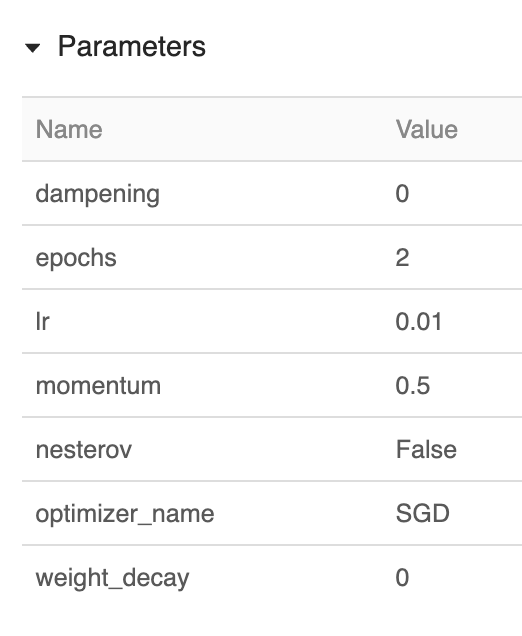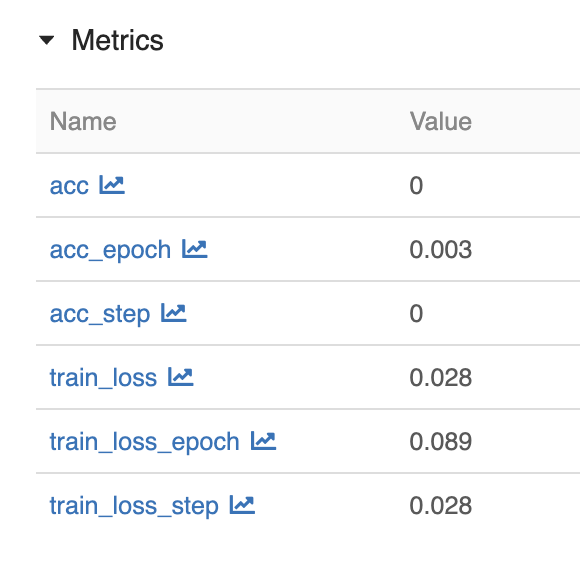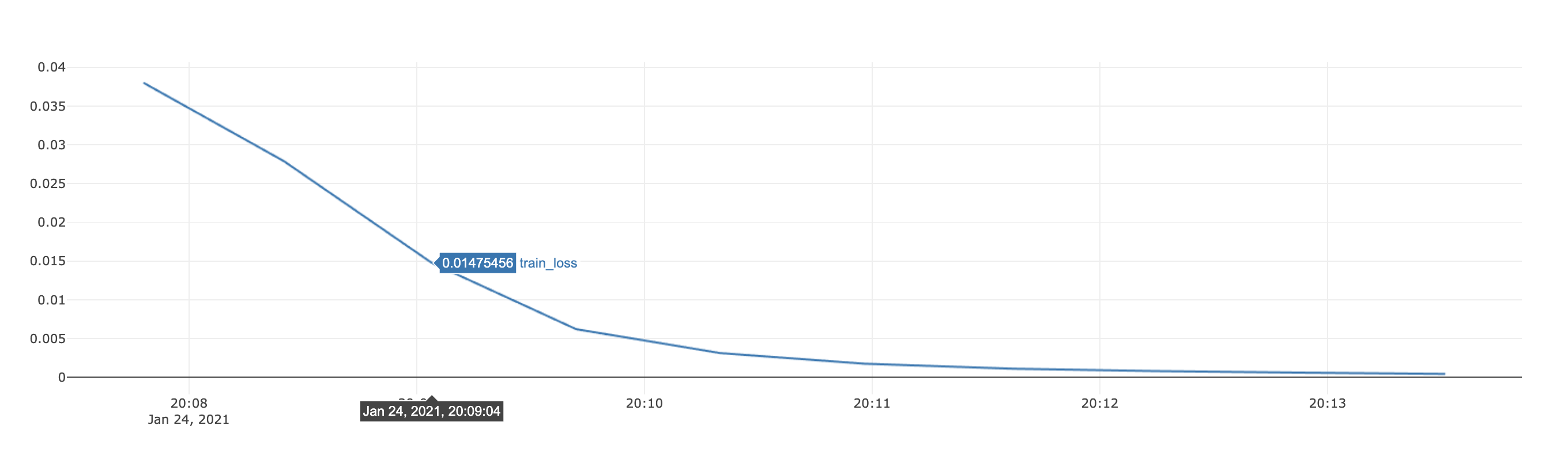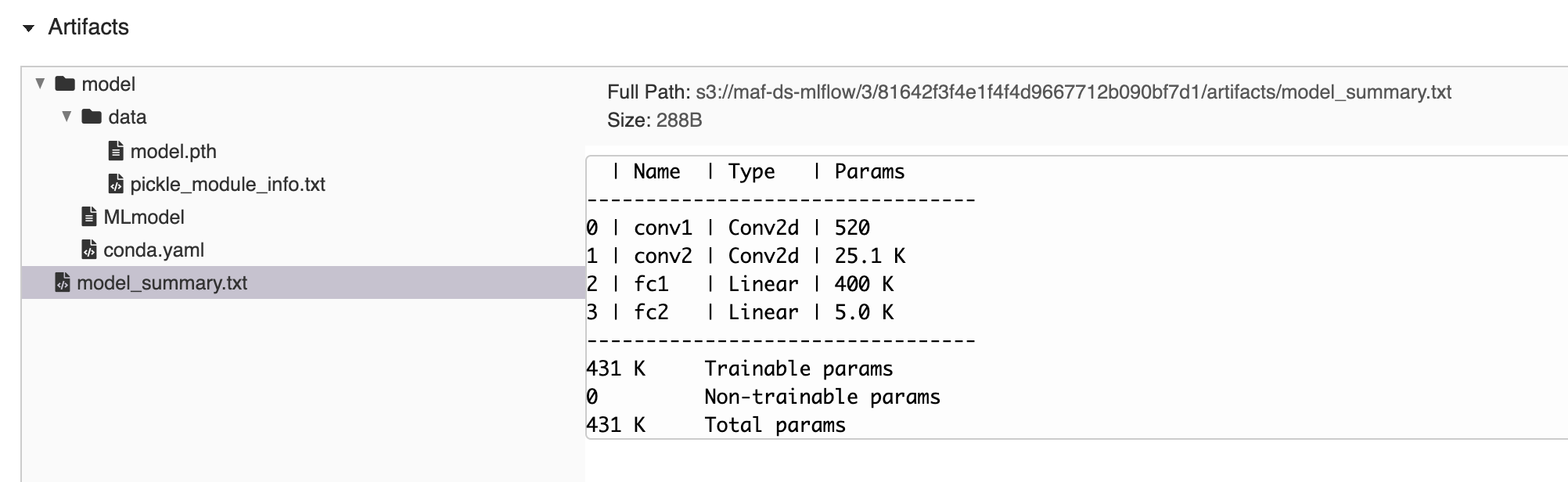# Automatic MLFlow logging for Pytorch

Marton Trencseni - Sun 24 January 2021 - Data

## Introduction

In a previous post I looked at getting MLFlow up and running and emitting some simple logs using log_artifact(), log_param(), log_metric(). Then I played around with automatic logging for Scikit Learn, and in the process even found some bugs in MLFlow, and fixed them. In this post, I will look at the automatic logging capabilities of MLFlow, but this time for Pytorch. The relevant documentation is here. The source code is up on Github.

## Autologging for classic Pytorch code

First, we have to turn on MLFlow itself and the autologging:

import mlflow
mlflow.set_tracking_uri('http://127.0.0.1:5000')  # set up connection
mlflow.set_experiment('test-experiment')          # set the experiment
mlflow.pytorch.autolog()


The easiest thing to try is to take existing Pytorch code, and run it after the above MLFlow code ran. So let's take the MNIST code from a previous blog post (only showing relevant bits):

class CNN(nn.Module):
def __init__(self):
...
def forward(self, x):
...

def train(model, device, train_loader, optimizer, epoch):
losses = []
model.train()
for batch_idx, (data, target) in enumerate(train_loader):
data, target = data.to(device), target.to(device)
output = model(data)
loss = F.nll_loss(output, target)
loss.backward()
optimizer.step()
losses.append(loss.item())
if batch_idx % 10 == 0:
print('Train Epoch: {} [{}/{} ({:.0f}%)]\tLoss: {:.6f}'.format(
100. * batch_idx / len(train_loader), loss.item()))
return losses

datasets.MNIST(...), batch_size=64, shuffle=True)
...
model = CNN()
optimizer = optim.SGD(model.parameters(), lr=0.01, momentum=0.5)
for epoch in range(0, 10):


However, this will not work! Nothing will show up in MLFlow after we run the above code.

Why not? This is classic Pytorch code, where we control the training loop, in the approx. 10 lines of code in train(). MLFlow can't do any automatic logging, because it doesn't know where we're at in the training (or testing), so it doesn't know when or how to measure and emit metrics. For this, we need to transform the code so it's closer to Scikit Learn's fit() and predict() pattern.

This is what Pytorch Lightning gives us! Alternatively, if you actually read the linked MLFlow for Pytorch documentation, it states that automatic logging only works with Pytorch Lighning 😊

## Autologging for Pytorch Lightning code

Let's refactor the above code to use Pytorch Lighning:

class MNISTModel(pl.LightningModule):
def __init__(self):
# same as in CNN
super(MNISTModel, self).__init__()
self.conv1 = nn.Conv2d(1, 20, 5, 1)
self.conv2 = nn.Conv2d(20, 50, 5, 1)
self.fc1 = nn.Linear(4*4*50, 500)
self.fc2 = nn.Linear(500, 10)

def forward(self, x):
# same as in CNN
x = F.relu(self.conv1(x))
x = F.max_pool2d(x, 2, 2)
x = F.relu(self.conv2(x))
x = F.max_pool2d(x, 2, 2)
x = x.view(-1, 4*4*50)
x = F.relu(self.fc1(x))
x = self.fc2(x)
return F.log_softmax(x, dim=1)

def training_step(self, batch, batch_nb):
x, y = batch
loss = F.nll_loss(self(x), y)
acc = accuracy(loss, y)
self.log("train_loss", loss, on_epoch=True)
self.log("acc", acc, on_epoch=True)
return loss

def configure_optimizers(self):

mnist_model = MNISTModel()
train_ds = MNIST(os.getcwd(), train=True,
trainer = pl.Trainer(max_epochs=2, progress_bar_refresh_rate=0)

with mlflow.start_run() as run:


In the Pytorch Lighning structure, it's now obvious when training starts and ends, and what a training step is. MLFlow autologging works. Note that logging of the metrics is not "free", we have to call self.log() in training_step(). This is because the framework doesn't know what kind of merics make sense for our model (eg. is it nll_loss() or cross_entropy()) and how to compute it.

So what gets logged? The parameters of the model:Various metrics, such as accuracy and loss are logged for each epoch:Showing loss per training epoch:Various artifacts, similar to the Scikit Learn case, such as the environment description and the pickled model:## Conclusion

The automatic logging capabilities are still experimental, but it's a great start. The logging only works with Pytorch Lighning, a great excuse to refactor classic Pytorch code to use Lighning. I will definitely turn on automatic logging for my production Pytorch (Lightning) models.

Happy MLFlowing!